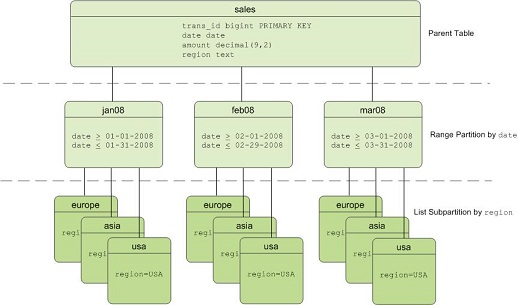## 支持的表分区类型

• 范围（RANGE）分区：基于一个数值型范围划分数据，例如按着日期区间定义。
• 值（LIST）分区：基于一个值列表划分数据，例如按着 城市属性定义。
• 多级分区表：上述两种类型的多级组合。## 创建范围(RANGE)分区表

``````CREATE TABLE sales (id int, date date, amt decimal(10,2))
DISTRIBUTED BY (id)
PARTITION BY RANGE (date)
( START (date '2016-01-01') INCLUSIVE
END (date '2017-01-01') EXCLUSIVE
EVERY (INTERVAL '1 day') );``````

``````CREATE TABLE rank (id int, rank int, year int, gender char(1), count int)
DISTRIBUTED BY (id)
PARTITION BY RANGE (year)
( START (2006) END (2016) EVERY (1),
DEFAULT PARTITION extra ); ``````

## 创建值（LIST )分区表

``````CREATE TABLE rank (id int, rank int, year int, gender
char(1), count int )
DISTRIBUTED BY (id)
PARTITION BY LIST (gender)
( PARTITION girls VALUES ('F'),
PARTITION boys VALUES ('M'),
DEFAULT PARTITION other );``````

## 创建多级分区表

``````CREATE TABLE sales
(id int, year int, month int, day int, region text)
DISTRIBUTED BY (id)
PARTITION BY RANGE (month)
SUBPARTITION BY LIST (region)
SUBPARTITION TEMPLATE (
SUBPARTITION usa VALUES ('usa'),
SUBPARTITION europe VALUES ('europe'),
SUBPARTITION asia VALUES ('asia'),
DEFAULT SUBPARTITION other_regions)
(START (1) END (13) EVERY (1),
DEFAULT PARTITION other_months );``````

## 分区表查询优化

AnalyticDB for PostgreSQL 支持分区表的分区裁剪功能，根据查询条件会只扫描所需的数据分区而避免扫描整个表的全部内容，提升查询性能。例如对于如下查询：
``````explain
select * from sales
where year = 2008
and  month = 1
and  day = 3
and region = 'usa';``````

``````Gather Motion 4:1  (slice1; segments: 4)  (cost=0.00..431.00 rows=1 width=24)
->  Sequence  (cost=0.00..431.00 rows=1 width=24)
->  Partition Selector for sales (dynamic scan id: 1)  (cost=10.00..100.00 rows=25 width=4)
Filter: year = 2008 AND month = 1 AND region = 'usa'::text
Partitions selected:  1 (out of 468)
->  Dynamic Table Scan on sales (dynamic scan id: 1)  (cost=0.00..431.00 rows=1 width=24)
Filter: year = 2008 AND month = 1 AND day = 3 AND region = 'usa'::text``````

## 查询分区表定义

``````SELECT
partitionboundary,
partitiontablename,
partitionname,
partitionlevel,
partitionrank
FROM pg_partitions
WHERE tablename='sales';``````